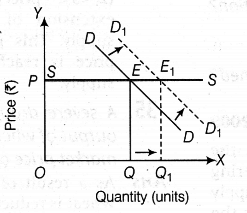# Define equilibrium price

Define equilibrium price. Show with the help of a diagram, the effect on equilibrium price when demand increases and supply is perfectly elastic.

Equilibrium price is that price at which quantity demanded is equal to quantity supplied. Given figure shows that equilibrium price will remain unchanged (OP only) despite increase in demand when supply is perfectly elastic.
When demand increases, equilibrium quantity increases from OQ to {{OQ}_{1}} but equilibrium -price remains constant (OP).